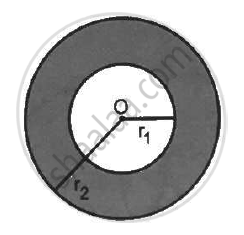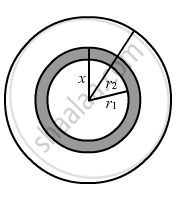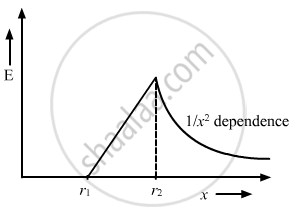Department of Pre-University Education, Karnataka course PUC Karnataka Science Class 12
Share

# A Charge Q is Distributed Uniformly Within the Material of a Hollow Sphere of Inner and Outer Radii R1 and R2 - Physics

ConceptElectric Field Introduction of Electric Field

#### Question

A charge Q is distributed uniformly within the material of a hollow sphere of inner and outer radii r1 and r2 (see the figure). Find the electric field at a point P at a distance x away from the centre for r1 < x < r. Draw a rough graph showing the electric field as a function of x for 0 < x < 2r2 (see the figure).#### Solution

Amount of charge present on the hollow sphere = Q

Inner radii of the hollow sphere = r1

Outer radii of the hollow sphere = r2

Consider an imaginary sphere of radius x.

The charge on the sphere can be found by multiplying the volume charge density of the hollow spherical volume with the volume of the imaginary sphere of radius (x-r1).

Charge per unit volume of the hollow sphere,

ρ = "Q"/( 4/3pi("r"_2^3 -"r"_1^3))

Charge enclosed by this imaginary sphere of radius x:-

q = ρ × Volume of the part consisting of charge

"q" = (4/3 pi ("x"^3 -"r"_1^3)"Q")/(4/3pi ("r"_2^3))

"q" = (("x"^3 -"r"_1^3))/(("r"_2^3 - "r"_1^3)) "Q"

According to Gauss's Law,

oint  "E" . "d""s" = "q"/∈_0

Here, the surface integral is carried out on the sphere of radius x and q is the charge enclosed by this sphere."E" oint "d""s" = "q"/∈_0

"E" (4 pi "x"^2) = "q"/∈_0

 "E" (4 pi "x"^2) = (("x"^3 -"r"_1^3)"Q")/((("r"_2^3 -"r"_1^3)) ∈_0)

"E"  =("Q"("x"^3 - "r"_1^3))/( 4pi ∈_0 "x"^2 ("r"_2^3 - "r"_1^3))

The electric field is directly proportional to x for r1 < x < r.

The electric field for r2 < x < 2r2,

"E"  = "Q" / (4 pi ∈_0 "x"^2)

Thus, the graph can be drawn as:-Is there an error in this question or solution?

#### APPEARS IN

Solution A Charge Q is Distributed Uniformly Within the Material of a Hollow Sphere of Inner and Outer Radii R1 and R2 Concept: Electric Field - Introduction of Electric Field.
S